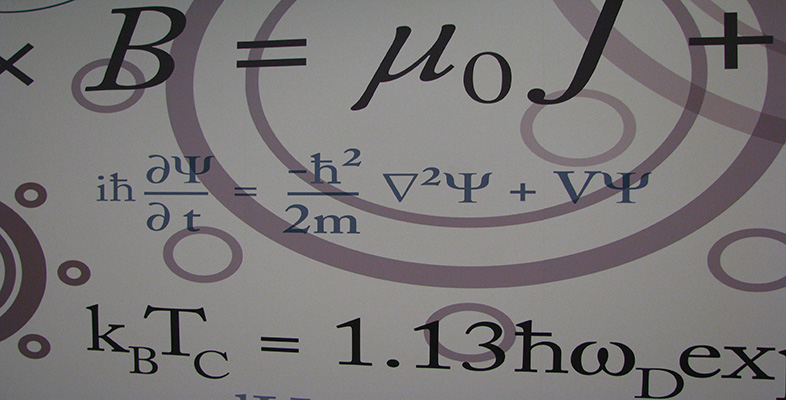Number systems

This free course is available to start right now. Review the full course description and key learning outcomes and create an account and enrol if you want a free statement of participation.

Free course

2 Complex numbers

2.1 What is a complex number?

We will now discuss complex numbers and their properties. We will show how they can be represented as points in the plane and state the Fundamental Theorem of Algebra: that any polynomial equation with complex coefficients has a solution which is a complex number. We will also define the function exp of a complex variable.

Earlier we mentioned several sets of numbers, including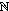,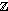,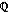and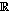. In each case, the numbers correspond to points on the real line. We now introduce numbers of a new kind, the so-called complex numbers, which correspond to points in the plane.

Complex numbers arise naturally as solutions of quadratic equations. For example, you showed in Exercise 3(e) that the equation x2 − 2x + 5 = 0 has no real solutions because there is no real number whose square is −16.

But suppose now that we ‘extend’ the set of real numbers by introducing a new number, denoted by i, which is defined to have the property that i2 = −1. Suppose further that i combines with itself, and with real numbers, according to the usual rules of arithmetic. In particular, assume that we can multiply i by any real number b to obtain the product ib, and that we can add on any real number a to obtain the sum a + ib. Such sums are known as complex numbers, and they are the numbers we need to solve the quadratic equation above.

We have ib = bi, and we can also write a + ib as a + bi.

Definitions

A complex number is an expression of the form x + iy, where x and y are real numbers and i2 = −1. The set of all complex numbers is denoted by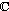.

A complex number z = x + iy has real part x and imaginary part y; we write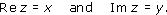Two complex numbers are equal when their real parts and their imaginary parts are equal.

For example, if z = 2 − 3i, then Re z = 2 and Im z = −3.

Remarks

1. Any given real number x can be written in the form x + i0, and any complex number of the form x + i0 is usually written simply as x. In this sense,is a subset of. The zero complex number 0 + i0 is written as 0.

2. We usually write a general complex number as x + iy, and a particular complex number as, for example, 2 + 3i, rather than 2 + i3. We write 2 − 3i rather than 2 + (−3)i.

3. Note that Re z and Im z are both real numbers.

4. A complex number of the form 0 + iy (where y ≠ 0) is sometimes called an imaginary number.

Since i2 = −1, we can regard the number i as a square root of −1. If we assume that the usual rules of arithmetic apply, then we can solve quadratic equations using the quadratic formula and the fact that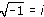. For example, consider the equation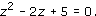This is the equation from Exercise 3(e), rewritten using z as the variable name. We often use the letter z for a complex variable (a variable that represents a complex number).

For the above equation, the quadratic formula gives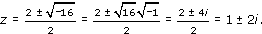We can check that these two complex numbers satisfy the equation we were trying to solve. We use the usual rules of arithmetic, and substitute −1 for i2 wherever it appears.

For example, if z = 1 + 2i, then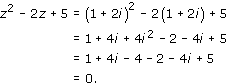4i2 = 4(−1) = −4, since i2 = −1.

The solution z = 1 − 2i can be checked similarly.

The use of the number i enables us to solve any quadratic equation in a similar way. We shall see later in the section that the use of i ensures that all polynomial equations have solutions, even those whose coefficients are themselves complex. This, in turn, means that any polynomial can be factorised into a product of linear factors; for example,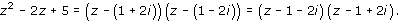Exercise 6

Solve the following equations, giving all solutions in.

• (a)  z2 − 4z + 7 = 0

• (b)  z2iz + 2 = 0

• (c)  z3 − 3z2 + 4z − 2 = 0 (Hint: z = 1 is one solution.)

• (d)  z4 − 16 = 0

Solution

• (a)  The equation z2 − 4z + 7 = 0 has solutions

•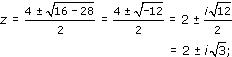• that is, z = 2 + i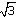and z = 2 − ii.

• (b)  The equation z2iz + 2 = 0 has solutions

•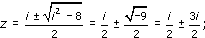• that is, z = 2i and z = − i.

• (c)  We can factorise the equation

•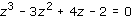• as

•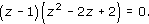• Hence z = 1 or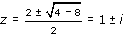,

• so the solutions are z = 1, z = 1 + i and z = 1 − i.

• (d)  z4− 16 = 0 can be factorised as

•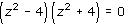• giving z2 = 4 or z2 = −4.

• Hence z = 2 or z = −2 or z =2i or z = −2i.

M208_6